# How to use the TYPE Function in Excel

The TYPE function returns numeric code representing the TYPE of data. It takes the value as an argument and returns the return TYPE of the value using the formula.
Syntax:

=TYPE (value)

Value : input value could be any value.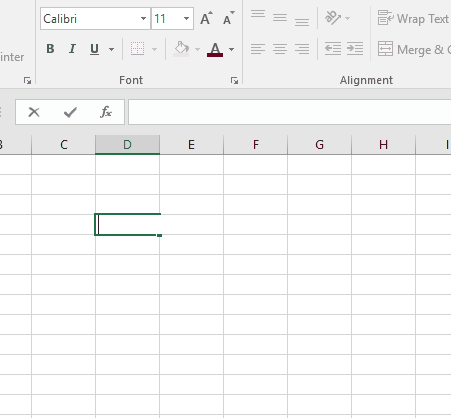Here the formula returns a number 2 which denotes that the value as an argument in the formula is TEXT.

 Return TYPE code Value TYPE 1 Number 2 Text 4 Logical value 16 Error 64 Array

Let’s understand this function using it in an example.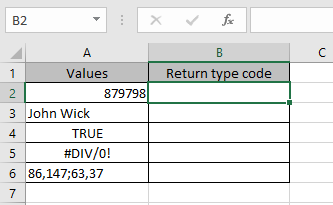Here we have different TYPEs of values for the function to return different return TYPEs.
Use the formula:

=TYPE(A2)

Note:
You can provide value to the function directly or as a cell reference.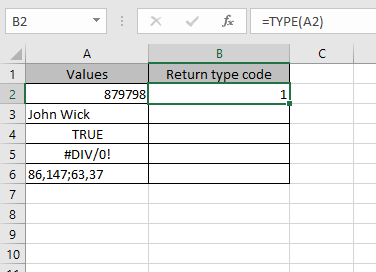Value is number, so the formula returns 1 as return type.
Copy the cells to the remaining cell using Ctrl + D shortcut key.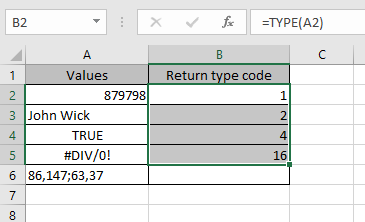As you can see the formula returns the correct TYPE of the data.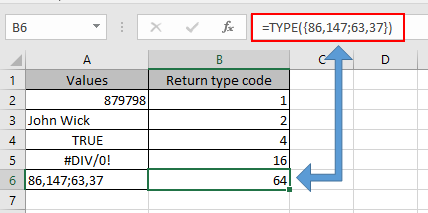But for the array, you need to give array in the argument directly to the formula.
You can get the return TYPE of value in text using the formula.
Use the formula to get the return TYPE of value in text:

=IF (TYPE(A2)=1, "Number", IF(TYPE(A2)=2,"Text", IF (TYPE(A2)=3, "Logical value", IF (TYPE(A2)=4, "Logical value", "Array"))))

Explanation:
The formula checks the value in TYPE function and if value is True, it returns the return TYPE in text.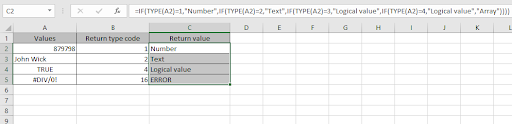As you can see we got all the return TYPE in text as required using the Excel formula.

Hope you understood how to use TYPE function and referring cell in Excel. Explore more articles on Excel functions here. Please feel free to state your query or feedback for the above article.

Related Articles

How to Use Nested IF Function in Excel

Using If Function to Compare Dates of Two Cells

The Excel IF Function

Popular Articles:

50 Excel Shortcuts to Increase Your Productivity

How to use the VLOOKUP Function in Excel

How to use the COUNTIF in Excel 2016

How to use the SUMIF Function in Excel

Edit a dropdown list

If with conditional formatting

If with wildcards

Vlookup by date

Terms and Conditions of use

The applications/code on this site are distributed as is and without warranties or liability. In no event shall the owner of the copyrights, or the authors of the applications/code be liable for any loss of profit, any problems or any damage resulting from the use or evaluation of the applications/code.